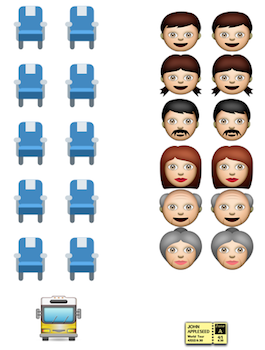Quantitative Finance

# Discrete Random Variables - Problem SolvingA commuter bus has $$10$$ seats. The probability that any passenger will not show up for the bus is $$0.6,$$ independent of other passengers. If the bus company sells $$12$$ tickets for the bus operation, what is the probability that at least one passenger will have to stand?

A parking building which is open for $$7$$ hours a day has the following fee policy: $$18$$ dollars per hour for the first 3 hours of parking, and $$6$$ dollars for each additional hour. Many years of data shows that the number of hours of parking for a car, denoted $$X,$$ is a discrete random variable with probability function $P(X = k) = \begin{cases} \frac{8 - k }{28}\ ( k = 1,2, \cdots, 7 ) \\ 0 \text{ otherwise.} \end{cases}$

What is the expected parking charge for a car in dollars under this policy?

Let $$X_1$$ and $$X_2$$ be random samples from a discrete distribution with probability function $P(X=k) = \begin{cases} \frac{1}{2} \quad ( k = 0 ) \\ \frac{1}{2} \quad ( k = 1 ). \end{cases}$ What is the expected value of $$X_1 \times X_2?$$

South Kingston High School, where James is attending, has a policy of giving discipline at weekend to those who were late for school in that week more than $$2$$ times. The probability that James is late for school is $$\frac{2}{13}.$$ The tardiness that occurs in any given day is independent of the tardiness that occurs in other days. What is the probability that James gets disciplined this weekend?

Note: James goes to school 5 days a week from Monday through Friday.

Kate and Devin are playing a game with two six-sided dice, each numbered 1 to 6. They each toss a die at the same time. If the product of the two numbers on the two dice is bigger than or equal to $$25,$$ Devin wins the game. What is the probability that Devin wins the game?

×